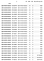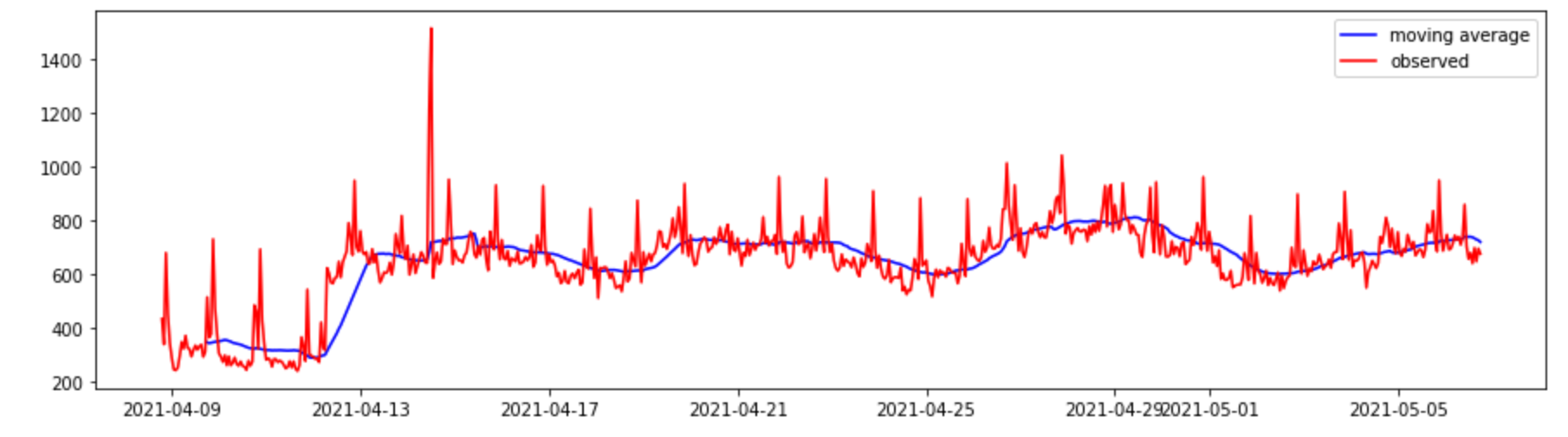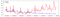# Time series forecasting with SARIMA

Previously I had looked into Facebook Prophet and this is now an attempt to learn moving average (MA) models and ARIMA. Wikipedia pages are not intuitive so this is an attempt to understand it and apply to the same server request rate example discussed previously.

# Moving Average

Moving average (also known as rolling average) at a given time in the time series is the mean of the previous N values. The N depends on the time series. For example, the given request rates data is hourly so using 24 values we can get the daily moving average.

`request_data['day_moving_avg'] = request_data.y.rolling(24).mean()`Daily moving averageObserved and moving average

## Moving Average Models

This looks simple? At each point you will notice a positive or negative difference between the MA and the observed value which is the error. So in the simplest model called MA(1) we use 1 error term coefficient to model it (reference youtube). Similarly you can think of MA(2) with 2 coefficients for a better fit and so on.

# SARIMA

Now recall the auto regressive (AR) approach where the PACF helps look at only specific prior points vs MA which relies on ACF. In the 1950s this was combined giving ARMA (reference). Trends and seasonality interfere with these models so ARIMA extracts the trend. I is “integrated” in this acronym (reference). And then there is SARIMA and S adds seasonality so SARIMA is what we really need in our case (reference). Using SARIMA requires a bunch of parameters and fortunately there is a pmdarima library to help with this “hyperparameter tuning”. You can refer to the notebook provided in the end for what I did but here are the predictions.SARIMA forecast vs observed

## More from Rahul Agarwal

https://linkedin.com/in/rahulaga